## Posts

Showing posts from July, 2019

### Hydrogen BondingHydrogen Bonding Nitrogen, oxygen and fluorine are the highly electronegative elements. When they are attached to a hydrogen atom to form covalent bond, the electrons of the covalent bond are shifted towards the more electronegative atom. This partially positively charged hydrogen atom forms a bond with the other more electronegative atom. This bond is known as hydrogen bond and is weaker than the covalent bond. For example, in HF molecule, the hydrogen bond exists between hydrogen atom of one molecule and fluorine atom of another molecule as depicted below:                                         ----H 𝛿 +       F 𝛿 - Here, hydrogen bond acts as a bridge between two atoms which holds one atom by covalent bond and the other by hydrogen bond. Hydrogen bond is represented by a dotted line (---) while a solid line represents the covalent bond. Thus, hydrogen bond can be defined as the attractive force which binds hydrogen atom of one molecule with the electronegative atom

### Conditions for the combination of atomic orbitals

Conditions for the combination of atomic orbitals The linear combination of atomic orbitals to form molecular orbitals takes place only if the following conditions are satisfied: 1. The combining atomic orbitals must have the same or nearly the same energy . This means that 1 s orbital can combine with another 1 s orbital but not with 2 s orbital because the energy of 2 s orbital is appreciably higher than that of 1 s orbital. This is not true if the atoms are very different. 2. The combining atomic orbitals must have the same symmetry about the molecular axis . By convention z -axis is taken as the molecular axis. It is important to note that atomic orbitals having same or nearly the same energy will not combine if they do not have the same symmetry. For example, 2 p z orbital of one atom can combine with 2 p z orbital of the other atom but not with the 2 p z or 2 p y orbitals because of their different symmetries. 3. The combining atomic orbitals must overla

### Linear Combination of Atomic Orbitals (LCAO)Linear Combination of Atomic Orbitals (LCAO) According to wave mechanics, the atomic orbitals can be expressed by wave functions (ψ ' s) which represent the amplitude of the electron waves. These are obtained from the solution of Schrodinger wave equation. However, since it cannot be solved for any system containing more than one electron, molecular orbitals which are one electron wave functions for molecules are difficult to obtained directly from the solution of Schrodinger wave equation. To overcome this problem, an approximate method known as linear combination of atomic orbitals (LCAO) has been adopted. Let us apply this method to the homonuclear diatomic hydrogen molecule. Consider the hydrogen molecule consisting of two atoms A and B. each hydrogen atom in the ground state has one electron in 1s orbital. The atomic orbitals of these atoms may be represented by the wave functions ψ A and ψ B . Mathematically, the formation of molecular orbitals may be described b

### Molecular Orbital Theory

Molecular Orbital Theory Molecular orbital (MO) theory was developed by F. Hund and R.S. Mulliken in 1932. The salient features of this theory are: The electrons in a molecule are present in the various molecular orbitals as the electrons of atoms are present in the various atomic orbitals. The atomic orbitals of camparable energies and proper symmetry combine to form molecular orbitals. While an electron in an aatomic orbital is influenced by one nucleus, in a molecular orbital it is influenced by two or more nuclei depending upon the number of atoms in the molecule. Thus, an atomic orbital is monocentric while a molecular orbital is polycentric. The number of molecular orbital formed is equal to the number of combining atomic orbitals. When two atomic orbitals combine, two molecular orbitals are formed. One is known as  bonding molecular orbital  while the other is called  antibonding molecular orbital . The bonding molecular orbital has lower energy and hence greater s

### sp3d2 Hybridizationsp 3 d 2 Hybridization In SF 6 the central sulphur atom has the ground state outer electronic configuration 3 s 2 3 p 4 . In the exited state the available six orbitals i.e ., one s , three p and two d are singly occupied by electrons. These orbitals hybridise to form six new sp 3 d 2 hybrid orbitals, which are projected towards the six corners of a regular octahedron in SF 6 . These six sp 3 d 2 hybrid orbitals overlap with singly occupied orbitals of fluorine atoms to form six S-F sigma bonds. Thus SF 6 molecule has a regular octahedral geometry as shown below

### sp3d Hybridization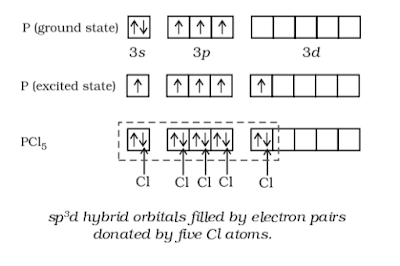sp 3 d Hybridization sp 3 d hybridization is shown in phosphorus penta chloride (PCl 5 ). The grounds state and the excited state outer electronic configurations of phosphorus (Z=15) are represented below. Now the five orbitals ( i.e ., one s , three p and one d orbitals) are available for hybridization to yield a set of five sp3d hybrid orbitals which are directed towards the five corners of a trigonal bipyramidal as depicted in the below.   It should be noted that tall the bond angles in trigonal bipyramidal geometry are not equivalent. In PCl 5 the five sp 3 d orbitals of phosphorus overlap with the singly occupied p orbitals of chlorine atoms to form five P-Cl sigma bonds. Three P-Cl bond lie in one plane and make an angle of 120 with each other; these bonds are termed as equatorial bonds. The remaining two P-Cl bonds-one lying above and the other lying below the equatorial plane, make an angle of 90 with the plane. These bonds are called axial bonds. As the

### C2H2 HybridizationC 2 H 2 Hybridization In the formation of ethyne molecule, both the carbon atoms undergo sp -hybridization having two unhybridized orbital i.e., 2 p y and 2 p x .   One sp hybrid orbital of one carbon atom overlaps axially with sp hybrid orbital of the other carbon atoms to form C-C sigma bonds. While the other hybridized orbital of each carbon atom overlaps axially with the half filled s orbital of hydrogen atoms forming   bonds. Each of the two unhybridised p orbitals of both the carbon atoms overlaps sidewise to form two  𝜋  bonds between the carbon atoms. so the triple bonds between the two carbon atoms is made up of one sigma and two pi bonds as shown in the below figure.

### C2H4 HybridizationC 2 H 4 Hybridization In the formation of ethene molecule, one of the sp 2 hybrid orbitals of carbon atom overlaps axially with sp 2 hybridized orbitals of another carbon atom to form C-C sigma bond. While the other two sp 2 hybrid orbitals of each carbon atom are used for making sp 2 -s sigma bond with two hydrogen atoms. The unhybridised orbital (2 p x or 2 p y ) of one carbon atom overlaps sidewise with the similar orbital of the other carbon atom to form weak  𝜋  bond, which consists of two equal electron clouds distributed above and below the plane of carbon and hydrogen atoms. Thus, in ethane molecule, the carbon-carbon bond consists of one sp 2 - sp 2 sigma bond and one pi (𝜋) bond between p orbitals which are not used in the hybridization and are perpendicular to the plane of molecule; the bond length 134 pm. The C-H bond is sp 2 - s sigma with bond length 108 pm. The H-C-H bond angle is 117.6 while the H-C-C angle is 121. The formation of sigma and pi bon

### Sp3 hybridization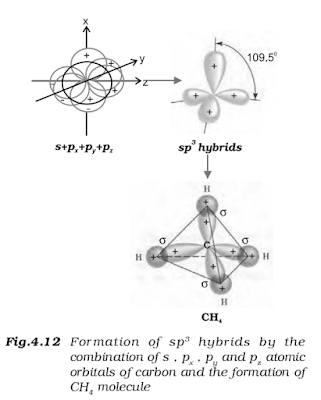Sp 3 hybridization This type of hybridization can be explained by taking the example of CH 4 molecule in which three is mixing of one s-orbital and three p -orbitals of the valence shell to form four sp 3 hybrid orbital of equivalent energies and shape. There is 25% s -character and 75% p -character in each sp 3 hybrid orbital. The four sp 3 hybrid orbitals so formed are directed towards the four corners of the tetrahedron. The angle between sp 3 hybrid orbital is 109.5 as shown below. The structure of NH 3 and H 2 O molecules can also be explained with the help of sp 3 hybridization.   In NH 3 , the valence shell (outer) electronic configuration of nitrogen in the grounds state is 2 s 2   2 p 1 x   2 p 1 y   2 p 1 z having three unpaired electrons in the sp 3 hybrid orbitals and a lone pair of electrons is present in the fourth one. These three hybrid orbitals overlap with 1 s orbitals of hydrogen atoms to form three N-H sigma bonds.   As we know that the f

### sp2 hybridizationsp 2 hybridization In this hybridization there is involvement of one s and two p-orbitals in order to form three equivalent sp 2 hybridized orbitals. For example, in BCl 3 molecule, the ground state electronic configuration of central boron atom is 1 s 2 2 s 2 2 p 1 . In the excited state, one of the 2 s electrons is promoted to vacant 2 p orbitals as a result boron has three unpaired electrons. These three orbitals (one 2 s and two 2 p ) hybridise to form three sp 2 hybrid orbitals. The three hybrid orbitals so formed are oriented in a trigonal planar arrangement and overlap with 2 p orbitals of chlorine to form three B-Cl bonds. Therefore, in BCl 3 the geometry is trigonal planar with CIBCl bond of 120.

### sp hybridization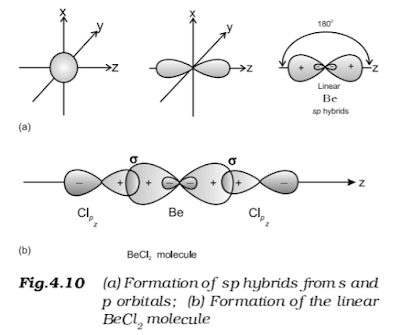sp hybridization This type of hybridization involves the mixing of one s and one p orbital resulting  in the formation of two equivalent sp hybrid orbitals. The suitable orbitals for sp hybridization are s and pz , if the hybrid orbitals are to lie along the z -axis. Each sp hybrid orbitals has 50% s -character and 50% p -character. Such a molecule in which the central atom is sp -hybridized and linked directly to two other central atoms possesses liner geometry. This type of hybridization is also known as diagonal hybridization. The tow sp hybrids point in the opposite direction along the z -axis with projecting positive lobes and very small negative lobes, which provides more effective overlapping resulting in the formation of stronger bonds. Example of molecule having sp hybridization BeCl 2 : The ground state electronic configuration of Be is 1 s 2 2 s 2 . In the exited state one of the 2 s -electrons is promoted to vacant 2p orbital to account for its divalenc

### Types of HybridizationTypes of Hybridization There are various types of hybridization involving s, p, and d orbitals. The different types of hybridization are as under. sp hybridization :  This type of hybridization involves the mixing of one s and one p orbital resulting   in the formation of two equivalent sp hybrid orbitals. The suitable orbitals for sp hybridization are s and pz , if the hybrid orbitals are to lie along the z -axis. Each sp hybrid orbitals has 50% s -character and 50% p -character. Such a molecule in which the central atom is sp -hybridized and linked directly to two other central atoms possesses liner geometry. This type of hybridization is also known as diagonal hybridization. The tow sp hybrids point in the opposite direction along the z -axis with projecting positive lobes and very small negative lobes, which provides more effective overlapping resulting in the formation of stronger bonds. Example of molecule having sp hybridization BeCl 2 : The ground state

### HybridizationHybridization In order to explain the characteristic geometrical shapes of polyatomic molecules like CH 4 , NH 3 and H 2 O etc., Pauling introduced the concept of hybridization. According to him the atomic orbitals combine to form new set of equivalent orbitals known as hybrid orbitals . Unlike pure orbitals, the hybrid orbitals are used in bond formation. The phenomenon is knows as hybridization which can be defined as the process of intermixing of the orbitals of slightly different energies so as to redistribute their energies, resulting in the formation of new set of orbitals of equivalent energies and shape. For example when one 2 s and three 2 p -orbitals of carbon hybridise, there is the formation of four new sp 3 hybrid orbitals. Salient features of hybridization: The main features of hybridization are as under: The number of hybrid orbitals is equal to the number of the atomic orbitals that get hybridized. The hybridized orbitals are always equivalent in e

### Pi (𝜋) bondPi (𝜋) bond It is a type of covalent bond. In the formation of  𝜋  bond the atomic orbitals overlap in such a way that there axes remain parallel to each other and perpendicular to the internuclear axis. The orbitals formed due to sidewise overlapping consists of two saucer type charged clouds above and below the plane of the participating atoms.

### Sigma (𝞂) bond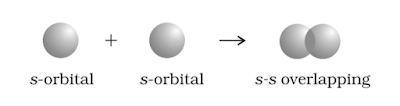Sigma (𝞂) bond This type of covalent bond is formed by the end to end (hand-on) overlap of bonding orbitals along the internuclear axis. This is called as head on overlap or axial overlap. This can be formed by any one of the following types of combinations of atomic orbitals. s-s overlapping : In this case, there is overlap of two half filled s-orbitals along the internuclear axis as shown below: s-p overlapping : This type of overlapping occurs between half filled s-orbitals of one atom and half filled p -orbitals of another atom. p-p overlapping : This type of overlap takes place between half filled p-orbitals of the two approaching atoms.

### Overlapping of Atomic OrbitalsOverlapping of Atomic Orbitals When two atoms come close to each other, there is overlapping of atomic orbitals. This overlap may be positive, negative or zero depending upon the properties of overlapping of atomic orbitals. The various arrangements of s and p orbitals resulting in positive, negative and zero overlap are depicted in below figure. The criterion of overlap, as the main factor for the formation of covalent bonds applies uniformly to the homonuclear/heteronuclear diatomic molecules and polyatomic molecules. In the case of polyatomic molecules like CH 4 , NH 3 and H 2 O, the VB theory has to account for their characteristic shapes as well. We know that the shapes of CH 4 , NH 3 and H 2 O molecules are tetrahedral, pyramidal and bent respectively. It would be therefore interesting to find out if these geometrical shapes can be explained in terms of the orbital overlaps. Let us first consider the CH 4 (methane) molecule. The electronic configuration of carb

### Valence Bond Theory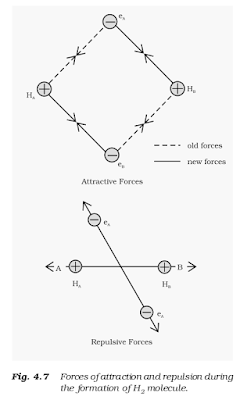Valence Bond Theory As we know the Lewis approach helps in writing the structure for molecules but it fails to explain the formation of chemical bond. It also does not give any reason for the difference in bond dissociation enthalpies and bond lengths in molecules like H 2 (435.8 kJ mol -1 , 74 pm) and F 2 (150.6 kJ mol -1 , 42 pm), although in both the cases a single covalent bond is formed by the sharing of an electron pair between the respective atoms. It also gives no idea about the shapes of polyatomic molecules. Similarly the VSEPR theory gives the geometry of simple molecules but theoretically, it does not explain them and also it has limited applications. To overcome these limitations the two important theories based on quantum mechanical principles are introduced. These are valence bond (VB) theory and molecular orbital (MO) theory. Valence bond theory was introduced by Heitler and London (1927) and developed further by Pauling and others. A discussion of the v

### VSEPR Theory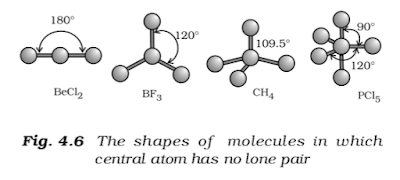VSEPR Theory Lewis concept is unable to explain the shapes of molecules. This theory provides a simple procedure to predict the shapes of covalent molecules. Sidgwick and Powell in 1940 proposed a simple theory based on the repulsive interactions of the electron pairs in the valence shell of the atoms. It was further developed and redefined by Nyholm and Gillespie (1957). The main postulates of VSEPR theory are as follows: The shape of a molecule depends upon the number of valence shell electron pairs (bonded or nonbonded) around the central atom. Pair of electrons in the valence shell repel one another since their electron clouds are negatively charged. These pairs of electrons tend to occupy such positions in space that minimize repulsion and thus maximize distance between them. The valence shell is taken as a sphere with the electron pairs localizing on the spherical surface at maximum distance from one another A multiple bond is treated as if it is a single electron p

### Polarity of BondPolarity of Bond The existence of a hundred percent ionic or covalent bond represents an ideal situation. In reality no bond or a compound is either completely covalent or ionic. Even in case of covalent bond between two hydrogen atoms, there is some ionic character. When covalent bond is formed between two similar atoms, for example in H 2 , O 2 , Cl 2 N 2 or F 2 , the shared pair of electrons is equally attracted by the two atoms. as a result electron pair is situated exactly between the two identical nuclei. The bond so formed is called nonpolar covalent bond. Contrary to this in case of a heteronuclear molecule like HF, the shared electron pair between the two atoms gets displaced more towards fluorine since the electronegativity of fluorine is far greater than that of hydrogen. The resultant covalent bond is a polar covalent bond. As a result of polarization, the molecule possesses the dipole moment (depicted below) which can be defined as the product of the magnitu